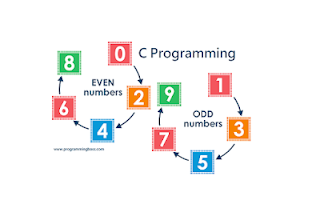# How to print odd numbers using c programming## Print all odd numbers using c programmingprint odd numbers using c programming

Before doing the code to find all odd numbers using c programming, we have to know the logic behind the legend. We have discussed the logical part of this post. Now it's time to write some code. But I Code to print all odd numbers using c programming. I suggest please go back to the logical part of the code first. The logical thinking to find odd and even number is the same.

int i, n, m;

First, define three integer variables. Then scan two integer values from the user using the scan function.

```printf("Minimum range: \n");
scanf("%d",&m);    printf("Maximum range: \n");    scanf("%d",&n);```

Then run a for loop to travel between the minimum and maximum range the user has given. And in the loop section, the statement will be used to determine whether the number is odd or even.

`for(i=m; i<=n; i++){            if(i%2!=0){                printf("%d\n",i);            }    } `

The complete code for printing all adds number in between given range is below:

Programming is all about practice. So, keep practicing and learn to program. I think now you know How to print all odd numbers using c programming in between a range given by the user.

--------------------- Happy Coding ------------------------